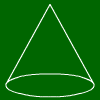# Cone calculation

For a cone, these formulae hold:
Base area = pi * Radius^2
Volume = 1/3 * base area * height
Lateral height = square root of (height^2+radius^2)
Surface = base area + lateral area

## What is a cone?A cone is a solid created in the following way: Take a circle and a point right over the center of that circle. Connect this point with the border of the circle, and you get a cone.

## What are formulas for cones?

These are the cone formulas: The base area equals Pi*r^2 (cause it is a circle). For the volume, the formula V=1/3*G*h holds, where G is the base area and h is the height.
For the distance between upper point and circle border, the formula s^2=h^2+r^2 holds (gotten from Pythagoras' theorem).
Last but not least, the furmula M=Pi*r*s gives the lateral area of the cone.

For further information, just move your mouse over one of the following words, and the corresponding piece of the cone will be marked.Radius, Perimeter, Base area,
height, lateral height
lateral area surface volume

## Cone calculation

Mathepower can calculate the base area, lateral area, surface, height and volume of a cone. Just enter your exercise and it will be solved step-by-step.